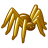PSoC™ 5, 3 & 1Level 1
Level 1

# what is the maximum streaming rate for Digital Filter Block

Hello,

I am having a 14 bit data stream from ADC and I want to use the DFB for FIR and IIR filters.

How do I find out what is the maximum data rate possible for using the DFB. In the technical reference manual @ pg 294, it is mentioned as

"A one 24-bit word Staging register is used for a sample rate at or below 1 Msps and guaranteed bus latency lower than the sample period." However in the creator it is not reporting any error for ADC sampling rate of 10MSPS.

In short please advise as to how do I find out information regarding the throughput for DFB.

1 SolutionLevel 9Level 9

# Re: what is the maximum streaming rate for Digital Filter Block

kuldeep,

The Filter needs some time to process sampled data so max sampling rate depends on filter depths and always less than bus_clk/10.

From Datasheet v.2.30 p. 4:

"The maximum possible sample rate is calculated by dividing the fastest available bus_clk by the number of DFB cycles that will be required by both filters. So, for each of the two channels, calculate a cycle count as follows:

FIR only:                                        10 + number_of_taps

Biquad even total order only:        13 + 5 × order

Biquad odd total order only:          18 + 5 × order

Both biquad even total order and FIR:      20 + number_of_taps + 5 × order

Both biquad odd total order and FIR:      25 + number_of_taps + 5 × order

Add together the results calculated for the two channels. This is the total number of bus_clk cycles that will be required to execute both channels. Dividing this into the bus_clk frequency will provide the value of the sample rate at which the faster of the two channels will run."

Secondly, where one can find a source of data for sampling at the rate higher than bus_clk/10? The DelSig-ADC can only provide <200kHz (@14-bit).

/odissey1Level 9Level 9

# Re: what is the maximum streaming rate for Digital Filter Block

kuldeep,

The Filter needs some time to process sampled data so max sampling rate depends on filter depths and always less than bus_clk/10.

From Datasheet v.2.30 p. 4:

"The maximum possible sample rate is calculated by dividing the fastest available bus_clk by the number of DFB cycles that will be required by both filters. So, for each of the two channels, calculate a cycle count as follows:

FIR only:                                        10 + number_of_taps

Biquad even total order only:        13 + 5 × order

Biquad odd total order only:          18 + 5 × order

Both biquad even total order and FIR:      20 + number_of_taps + 5 × order

Both biquad odd total order and FIR:      25 + number_of_taps + 5 × order

Add together the results calculated for the two channels. This is the total number of bus_clk cycles that will be required to execute both channels. Dividing this into the bus_clk frequency will provide the value of the sample rate at which the faster of the two channels will run."

Secondly, where one can find a source of data for sampling at the rate higher than bus_clk/10? The DelSig-ADC can only provide <200kHz (@14-bit).

/odissey1